Constructing Perpendicular bisector

Practical Geometry
Serial order wise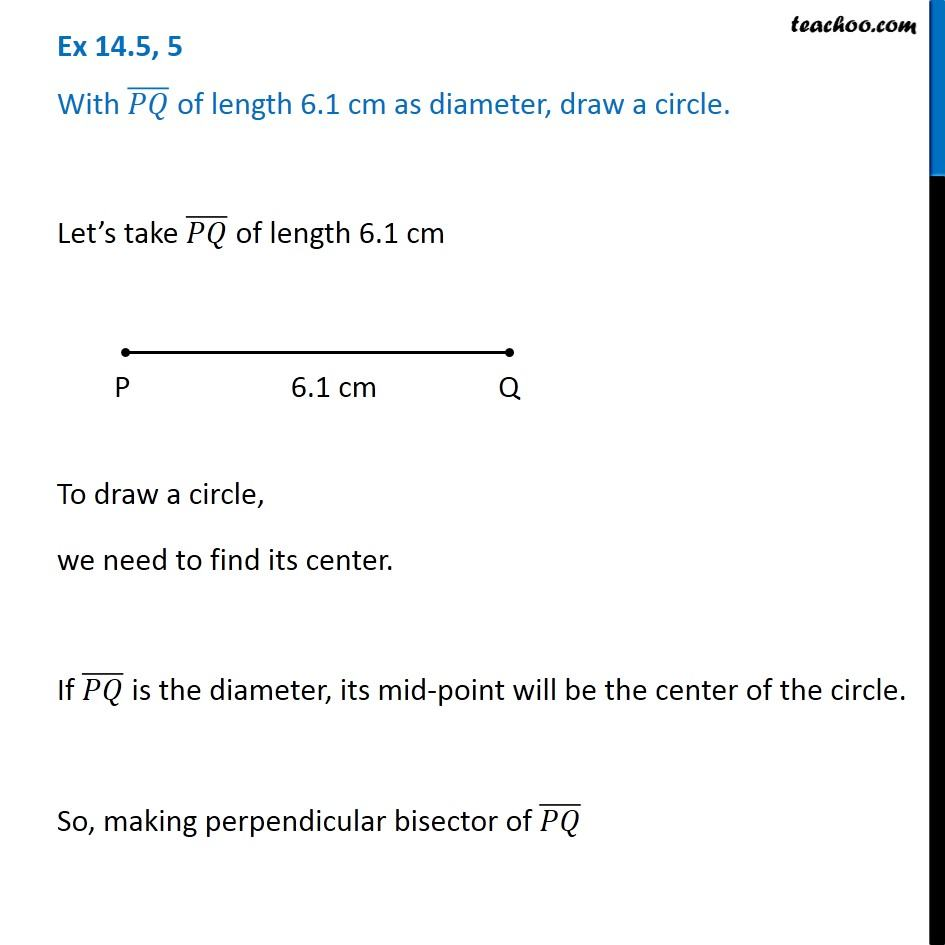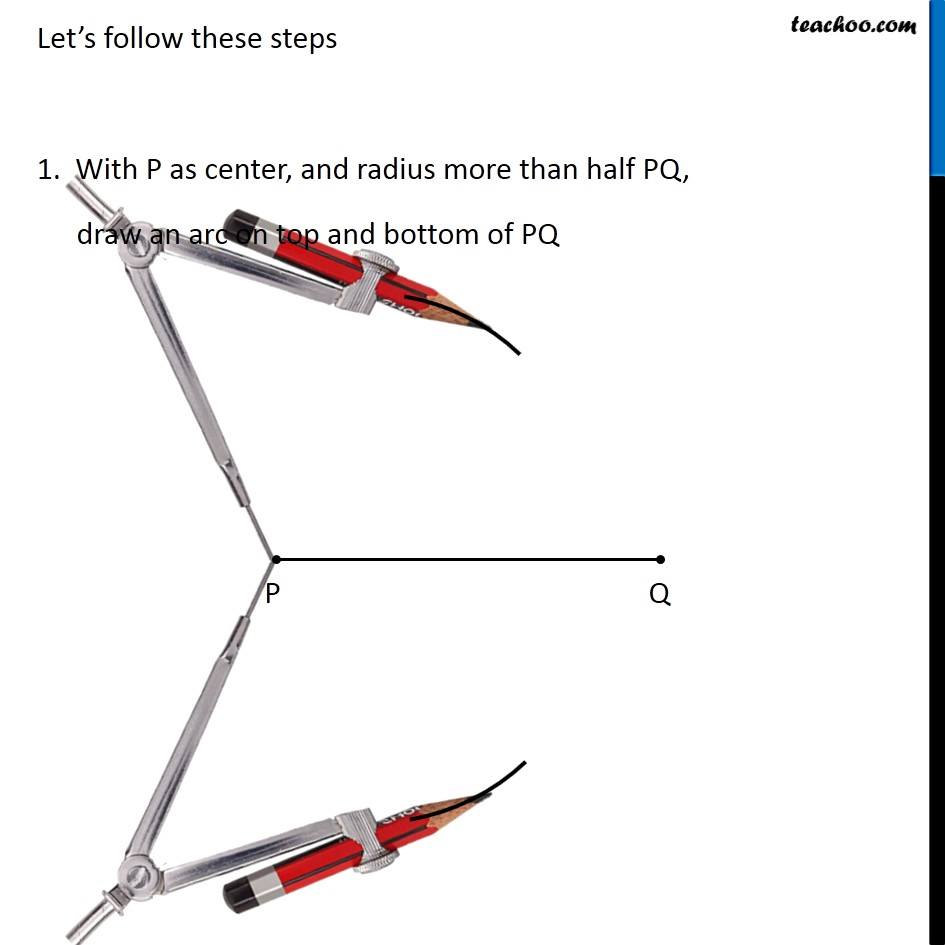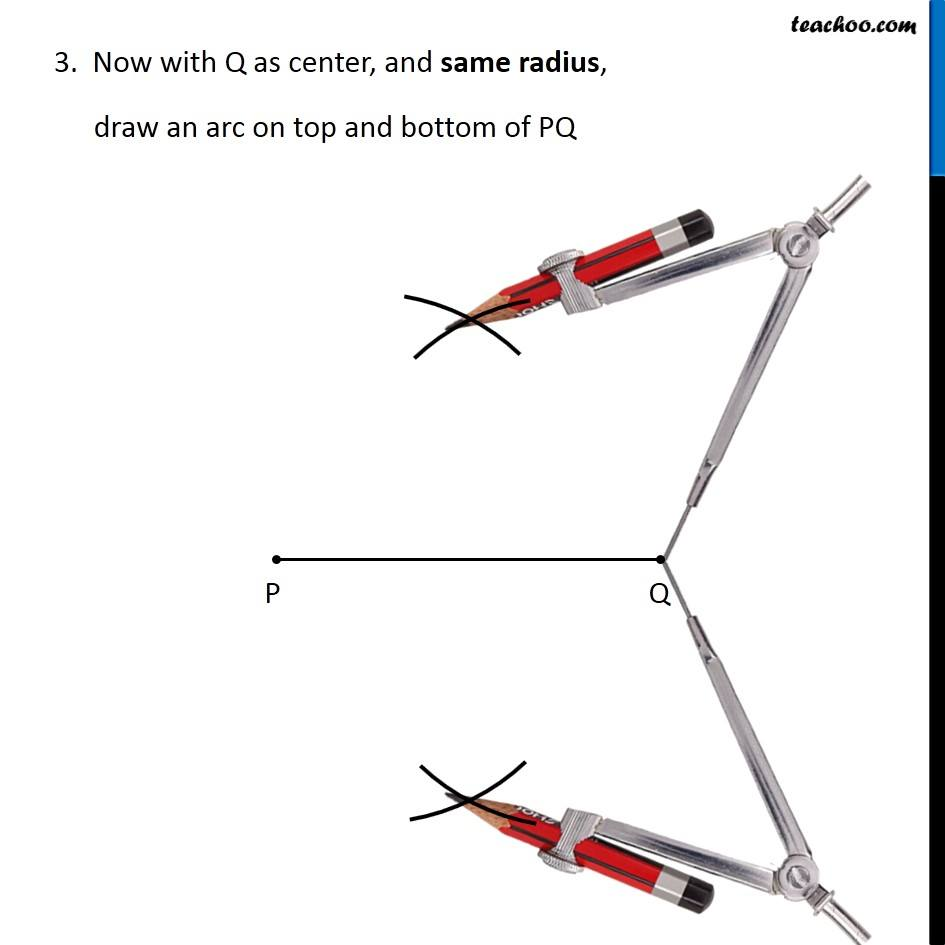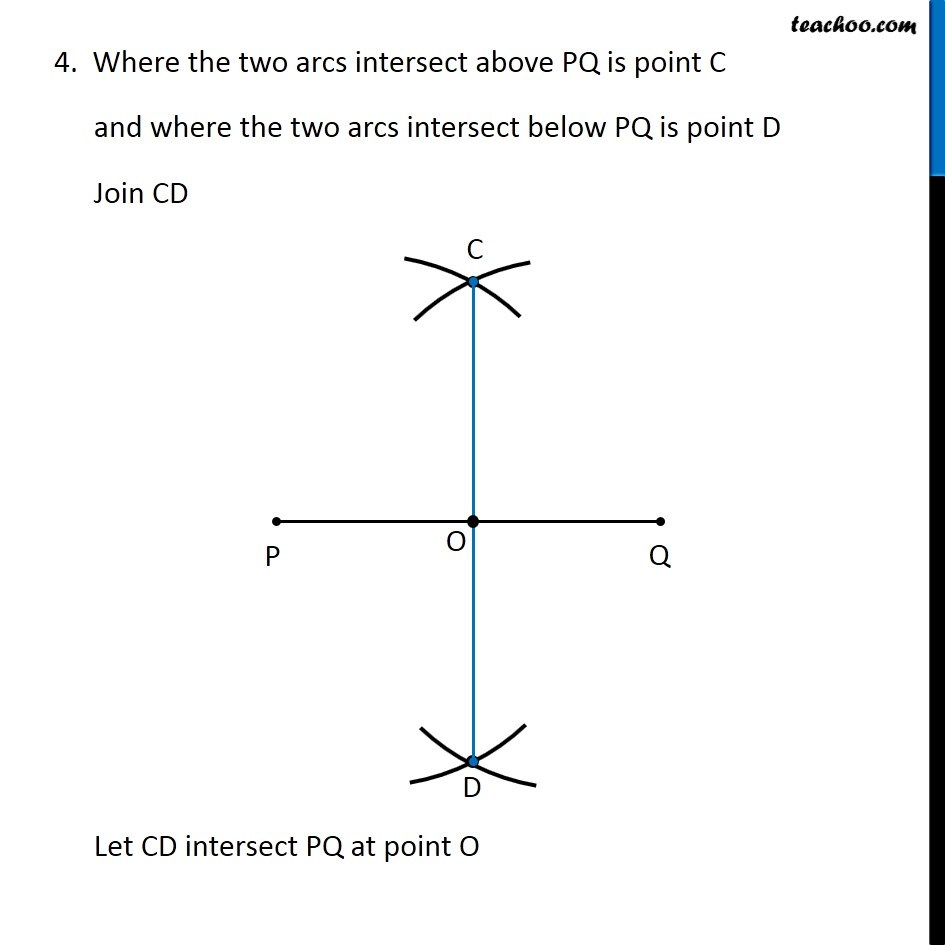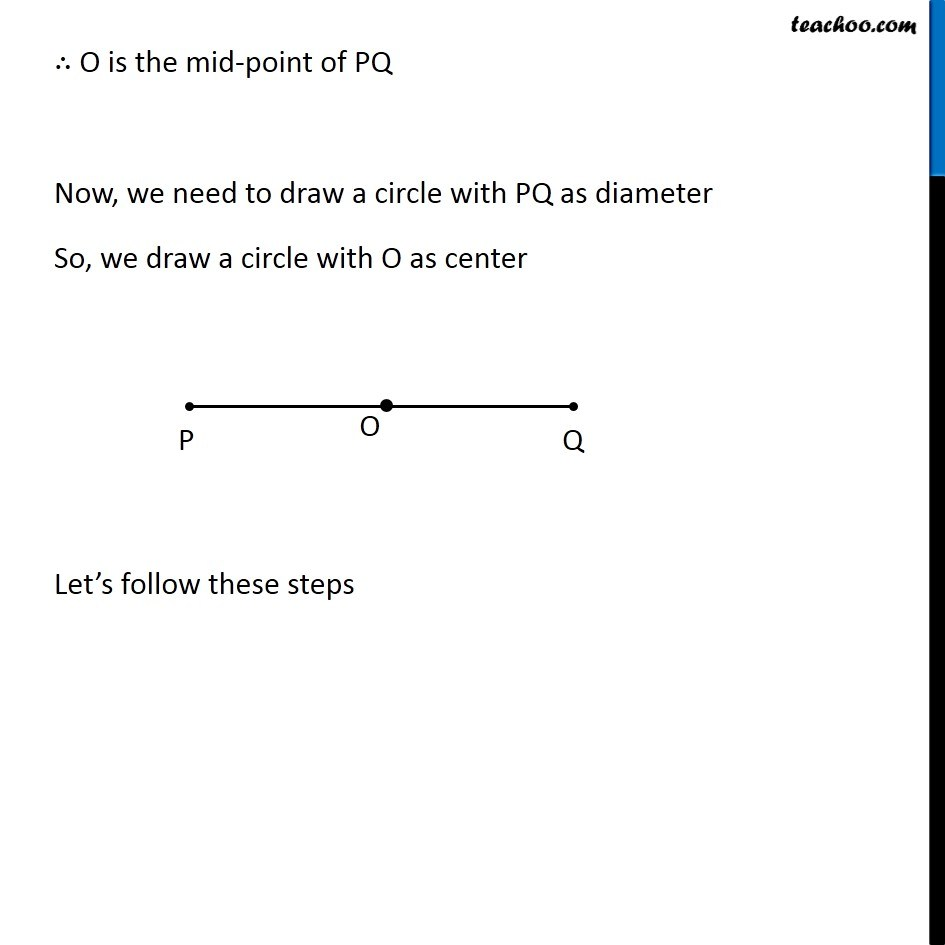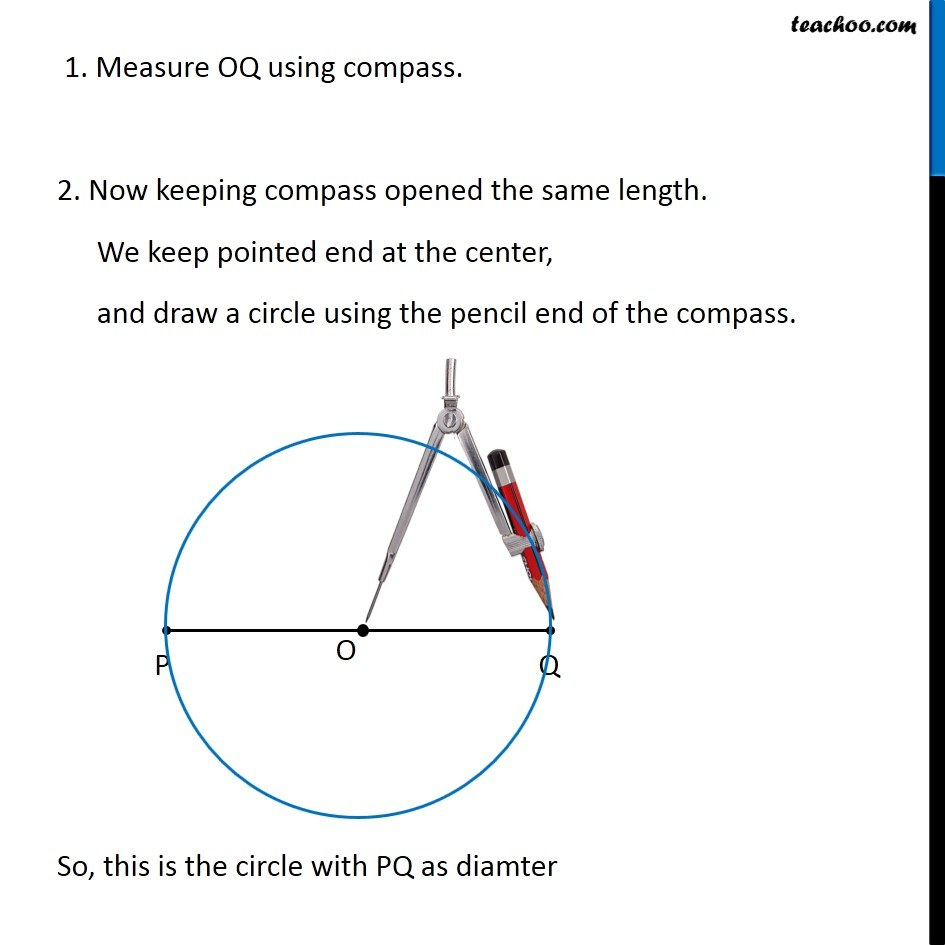Learn in your speed, with individual attention - Teachoo Maths 1-on-1 Class

### Transcript

Question 5 With (𝑃𝑄) ̅ of length 6.1 cm as diameter, draw a circle. Let’s take (𝑃𝑄) ̅ of length 6.1 cm To draw a circle, we need to find its center. If (𝑃𝑄) ̅ is the diameter, its mid-point will be the center of the circle. So, making perpendicular bisector of (𝑃𝑄) ̅ Let’s follow these steps 1. With P as center, and radius more than half PQ, draw an arc on top and bottom of PQ 3. Now with Q as center, and same radius, draw an arc on top and bottom of PQ 4. Where the two arcs intersect above PQ is point C and where the two arcs intersect below PQ is point D Join CD Let CD intersect PQ at point O ∴ O is the mid-point of PQ Now, we need to draw a circle with PQ as diameter So, we draw a circle with O as center Let’s follow these steps 1. Measure OQ using compass. 2. Now keeping compass opened the same length. We keep pointed end at the center, and draw a circle using the pencil end of the compass. So, this is the circle with PQ as diamter